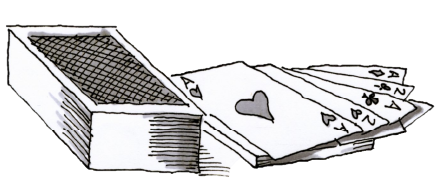### Home > CCG > Chapter 2 > Lesson 2.2.4 > Problem2-98

2-98.

What is the probability of drawing each of the following cards from a standard playing deck? Refer to the glossary entry “playing cards” if you need information about a deck of cards.There are $52$ cards in a deck.
Red: diamonds and hearts
$4$ of each: $\text{A}, 2, 3, 4, 5, 6, 7, 8, 9, 10,\text{J, Q, K}$

1. P(face card)

$\frac{12}{52}=\frac{3}{13}$

1. P(card printed with an even number)

$\frac{20}{52}=\frac{5}{13}$

1. P(red ace)

The Ace of diamonds and the Ace of hearts.

1. P(purple card)

There are no purple cards in a deck.